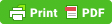﻿ Medical Impedance Meters# Medical Impedance Meters

Feed by sandy Cat- Health & Beauty

Rush et al  used a 50 kHz BIM4 Impedimed (Capalaba, Australia) with self-adhesive electrodes on Asian and Fijian Indians (110 men and 101 women) and developed a prediction equation for FFM based on BIA measurements. People who were diabetic, pregnant or taking medication afecting weight were excluded. They compared their FFM data with those of a GE Lunar DXA (Madison, WI, USA) by Student T-test, using nine equations from the BIA literature. hey provided mean +SD data for men and women, but they also separated subjects data in 5 columns by age 20–29 yr, 30–39, 40–49, 50–59 and 60+. Rush et al proposed two new BIA equations for FFM prediction, suited for male and female Asian Indian population. For 110 males, the equation was

FFM = 0.382 H2/R+0.167W+0.320H-33.382                                                              (1a)

where H is the height in cm, R the body resistance in ohm, and W the weight in kg. The correlation coincident was R2=0.84. Predicted mean FFM was 50.50±6.38 kg, against a DXA FFM of 50.44 ±3.38 kg and p value of T test was 0.52.

For 101 females, it was

FFM = 0.456H2/R+0.127W+0.0746X+5.959                                                              (1b)

where X is the reactance and R2= 0.70. Deta. P-values of Student T-test indicate whether differences are significant when p< 0.05 and not significant if p>0.05. A p-value of

0.68 indicates that differences are very small. Minimum bias was -0.42 kg with Bhat et al equation  in validation group for males

FFM= 0.287H2/Z +0.364W+12.97

It gave a mean FFM prediction of 50.0 ± 5.6 kg with a p-value of 0.22, which was close to DXA value of 50.18, very close to that of DXA. And for females, the minimum bias was 0.53 kg using Duremberg equation  in validation group.

FFM= 0.34H2/R +0.1534H+0.273W -0.127 Age+ 4.56 sex -12.44                              (2b)

The mean prediction was 35.73 ± 34 kg against a DXA value of 35.22 ± 2.79 kg and a p-value of 0.08, worse than for males.

Predicted mean FFM in women was 36.05 ± 3.0 kg versus 36.04 ± 3.60 kg by DXA and p= 0.33. he male equation was more accurate than the female one as its value of p was higher. he good results of Eqs 1a and 1b are due to the fact the entire cohorts were used to determine these equations. It is remarkable that BIA equations gave very close data for mean FFM and FM with DXA data for both men and women, although Asian Indians have higher body fat than Caucasian subjects. Only four of 101 women had %BF less than 30% and 17 men had %BF less than 25%. his is due to speciic equations developed by the authors.